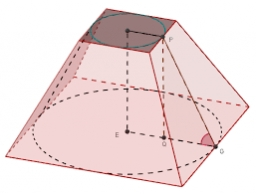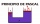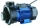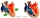# Runcated pyramid teapot

The 35 cm high teapot has the shape of a truncated pyramid with the length of the edge of the lower square base a=50 cm and with the edges of the rectangular base b: 20 cm and c: 30 cm. How many liters of water will fit in the teapot?

Correct result:

V =  50.4554 l

#### Solution:We would be pleased if you find an error in the word problem, spelling mistakes, or inaccuracies and send it to us. Thank you!Tips to related online calculators
Do you know the volume and unit volume, and want to convert volume units?

## Next similar math problems:

• The Chemistry testThe Chemistry test contained 8 questions, each with 3 points. Peter scored 21 points. How many percent did Peter write a test?
• Equations - simpleSolve system of linear equations: x-2y=6 3x+2y=4
• Art schoolEvery fifth pupil 9A goes to art school. How many percent of pupils in class 9A go to art school?
• Three brothersThe three brothers have a total of 42 years. Jan is five years younger than Peter and Peter is 2 years younger than Michael. How many years has each of them?
• Pascal's lawPlease calculate according to Pascal's law. Krupp's machines were known for their large size. In 1861, a blacksmith's steam hydraulic press was put into operation in Essen. What was the cross-sectional area of the larger piston if a compressive force of 1
• Cylindrical tank 2If a cylindrical tank with volume is used 12320cm raised to the power of 3 and base 28cm is used to store water. How many liters of water can it hold?
• RainfallThe annual average rainfall in India was in Cherrapunji in the year 1981 26 461 mm. How many hectoliters of water fell on 1 m2? Would fit this amount of water into a cube of three meters?
• Ice cream in coneIn the ice cream cone with a diameter of 5.7 cm is 0.8 dl of ice cream. Calculate the depth of the cone.
• GasholderThe gasholder has spherical shape with a diameter 23 m. How many m3 can hold in?
• Three pumps togetherOne pump fills the tank in 1.5 hours, the second in 2 hours, and the third in 3 hours 20 minutes. How many minutes will the tank fill with three pumps if they work simultaneously?
• An oilAn oil drum is cut in half. One half is used as a water trough. Use the dimensions; length 82cm, width 56cm to estimate the capacity of the water trough in liters.
• Permille of alcoholI have 2 per mille of alcohol in my blood. How many milliliters is it when I have 5 liters of blood?
• DiggingA pit is dug in the shape of a cuboid with dimensions 10mX8mX3m. The earth taken out is spread evenly on a rectangular plot of land with dimensions 40m X 30m. What is the increase in the level of the plot ?
• TrayWjat height reach water level in the tray shaped a cuboid, if it is 420 liters of water and bottom dimensions are 120 cm and 70 cm.
• Water levelHow high reaches the water in the cylindrical barell with a diameter of 12 cm if there is a liter of water? Express in cm with an accuracy of 1 decimal place.
• Conserving waterCalculate how many euros are spent annually on unnecessary domestic hot water, which cools during the night in pipeline. Residential house has 129 m of hot water pipelines 5/8" and the hot water has a price of 7 Eur/m3.
• Water wellDrilled well has a depth 20 meters and 0.1 meters radius. How many liters of water can fit into the well?